## Example Questions

### Example Question #11 : Physics

A particle accelerator functions by exerting a magnetic field on charged particles which are shot into the accelerator. The magnetic field causes the charged particles to move around in a circle of radiusthat can be predicted by the following equation, where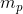is the mass of the particle in kilograms,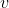is the initial speed at which the particle was shot in meters per second,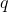is the charge of the particle in Coulombs, andis the strength of the magnetic field in Tesla.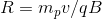A proton weighs approximatelyamu (atomic mass units) and has a charge of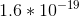C. An electron has the same magnitude of charge, but it has about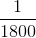of the proton's mass. What would happen to radiusif we were to suddenly switch the particle in the accelerator from a proton to an electron, keeping all of the other conditions the same?

Radiuswould increase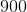-fold.

Radiuswould not change.

Radiuswould increase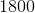-fold.

Radiuswould decrease-fold.

Radiuswould decrease-fold.

Radiuswould increase-fold.

Explanation:

The correct answer is that radiuswould decrease-fold. With a smaller mass, we know by the given equation that the radius would change in proportion with the change in particle mass. Therefore, if the mass decreased-fold, so would radius.

### Example Question #12 : Physics

Sound waves travel through a medium by mechanically disturbing the particles of that medium. As particles in the medium are displaced by the sound wave, they in turn act upon neighboring particles. In this fashion, the wave travels through the medium through a parallel series of disturbed particles. Like in other forms of motion, the rate at which the sound wave travels can be measured by dividing the distance over which the wave travels by the time required for it to do so.

Study 1
A group of students hypothesizes that the velocity of sound is dependent upon the density of the medium through which it passes. They propose that with more matter in a given space, each particle needs to travel a shorter distance to disturb the adjacent particles. Using two microphones and a high speed recording device, the students measured the delay from the first microphone to the second. They chose a variety of media, shown in Table 1, and measured the velocity of sound through each using their two-microphone setup. The results are found in Table 1.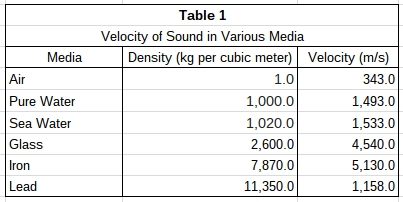Study 2
The students wanted to test their hypothesis by using the same medium at different densities. To do this, they heated pure water to various temperatures and repeated the procedure described in Study 1. Their results can be found in Table 2.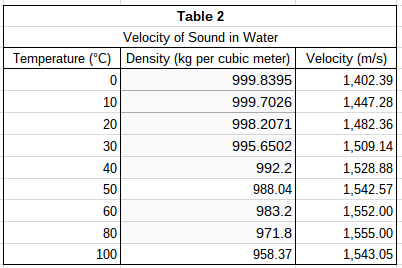If the temperature of iron were raised slightly in Study 1, what would be the most likely result?

Velocity would increase.

Velocity would decrease.

Velocity would remain constant.

Density would increase.

Velocity would increase.

Explanation:

Based on the results of Study 2, one can infer that increases in temperature tend to cause density to decrease and velocity to increase. Because this is the only temperature information in the passage, it is the best predictor of the effect of temperature change in iron.

### Example Question #13 : Physics

Sound waves travel through a medium by mechanically disturbing the particles of that medium. As particles in the medium are displaced by the sound wave, they in turn act upon neighboring particles. In this fashion, the wave travels through the medium through a parallel series of disturbed particles. Like in other forms of motion, the rate at which the sound wave travels can be measured by dividing the distance over which the wave travels by the time required for it to do so.

Study 1
A group of students hypothesizes that the velocity of sound is dependent upon the density of the medium through which it passes. They propose that with more matter in a given space, each particle needs to travel a shorter distance to disturb the adjacent particles. Using two microphones and a high speed recording device, the students measured the delay from the first microphone to the second. They chose a variety of media, shown in Table 1, and measured the velocity of sound through each using their two-microphone setup. The results are found in Table 1.Study 2
The students wanted to test their hypothesis by using the same medium at different densities. To do this, they heated pure water to various temperatures and repeated the procedure described in Study 1. Their results can be found in Table 2.Which study provides stronger evidence against the students' prediction and why?

Study 1, because using different media shows that velocity increases as density increases

Study 1, because using different media shows that velocity increases as density decreases

Study 2, because using the same medium shows that velocity decreases as density decreases

Study 2, because using the same medium shows that velocity increases as density decreases

Study 2, because using the same medium shows that velocity increases as density decreases

Explanation:

The students in the passage hypothesized that increased density would result in increased velocity of sound through that medium. Study 2 provides the most evidence to the contrary by showing decreasing densities linked to increasing velocities.

### Example Question #14 : Physics

Sound waves travel through a medium by mechanically disturbing the particles of that medium. As particles in the medium are displaced by the sound wave, they in turn act upon neighboring particles. In this fashion, the wave travels through the medium through a parallel series of disturbed particles. Like in other forms of motion, the rate at which the sound wave travels can be measured by dividing the distance over which the wave travels by the time required for it to do so.

Study 1
A group of students hypothesizes that the velocity of sound is dependent upon the density of the medium through which it passes. They propose that with more matter in a given space, each particle needs to travel a shorter distance to disturb the adjacent particles. Using two microphones and a high speed recording device, the students measured the delay from the first microphone to the second. They chose a variety of media, shown in Table 1, and measured the velocity of sound through each using their two-microphone setup. The results are found in Table 1.Study 2
The students wanted to test their hypothesis by using the same medium at different densities. To do this, they heated pure water to various temperatures and repeated the procedure described in Study 1. Their results can be found in Table 2.According to the information in the passage, what is necessary for sound to travel through a medium?

A medium in the gaseous state

Particles close enough to physically displace one another

Controlled temperatures

Two microphones and a high speed recording device

Particles close enough to physically displace one another

Explanation:

The passage states that sound waves travel by mechanically disturbing particles. Without a medium with particles close enough to interact physically, there would be no transfer of mechanical energy through the medium.

The remaining answers list conditions of the studies used to test the students' hypothesis about density and sound velocity. These are not essential for sound to travel through a medium.

### Example Question #15 : Physics

Sound waves travel through a medium by mechanically disturbing the particles of that medium. As particles in the medium are displaced by the sound wave, they in turn act upon neighboring particles. In this fashion, the wave travels through the medium through a parallel series of disturbed particles. Like in other forms of motion, the rate at which the sound wave travels can be measured by dividing the distance over which the wave travels by the time required for it to do so.

Study 1
A group of students hypothesizes that the velocity of sound is dependent upon the density of the medium through which it passes. They propose that with more matter in a given space, each particle needs to travel a shorter distance to disturb the adjacent particles. Using two microphones and a high speed recording device, the students measured the delay from the first microphone to the second. They chose a variety of media, shown in Table 1, and measured the velocity of sound through each using their two-microphone setup. The results are found in Table 1.Study 2
The students wanted to test their hypothesis by using the same medium at different densities. To do this, they heated pure water to various temperatures and repeated the procedure described in Study 1. Their results can be found in Table 2.In Study 1, if the students were to double the length of the samples of media, what would happen to the velocity of sound through those media?

It would decrease.

It would remain constant.

It would increase.

It would remain constant.

Explanation:

According to the passage, velocity of sound is found by dividing distance by time. Any increase in distance traveled would cause the time required for travel to increase proportionally. In this manner, velocity would remain constant if the students were to double the length of media samples in Study 1.

### Example Question #11 : How To Find Research Summary In Physics

A physicist wishes to study the trajectory of a ball launched horizontally. She varies parameters such as the launching velocity, starting height, and mass of the ball. For each trajectory, she records the time of flight (in seconds) and horizontal displacement (in meters). She assumes air resistance is negligible.

Figure 1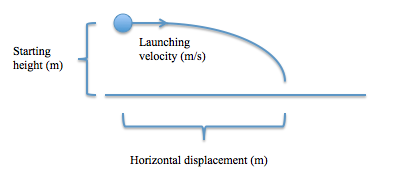Using all of the data she collects, she constructs the following table:

Table 1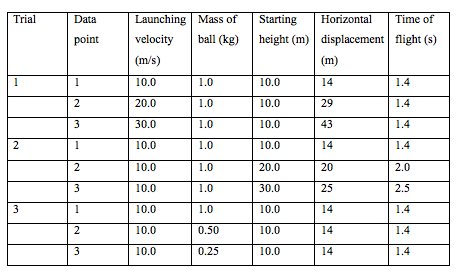Based on the data presented in the table, what appears to be the relationship between the mass of the ball and the time of flight?

The time of flight increases as the mass of the ball increases.

The time of flight is not affected by the mass of the ball.

The time of flight decreases as the mass of the ball increases.

Cannot be determined

The time of flight is not affected by the mass of the ball.

Explanation:

In trial 3, the time of flight remains the same as the mass of the ball is varied.

### Example Question #11 : Physics

A physicist wishes to study the trajectory of a ball launched horizontally. She varies parameters such as the launching velocity, starting height, and mass of the ball. For each trajectory, she records the time of flight (in seconds) and horizontal displacement (in meters). She assumes air resistance is negligible.

Figure 1Using all of the data she collects, she constructs the following table:

Table 1In which trial(s) did the time of flight change between data points?

Trial 3 only

Trial 2 only

Trials 2 and 3

Trials 1 and 2

Trial 2 only

Explanation:

By inspecting the table, we see that the time of flight changed between data points for Trial 2 only.

### Example Question #18 : Physics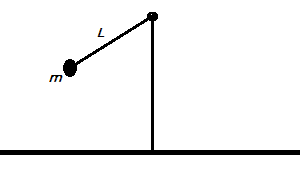The period of a simple pendulum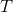is defined as the amount of time that it takes for a pendulum to swing from one end to the other and back. In studying the period of a simple pendulum, two students express their opinions.

Student 1: The period of a pendulum depends on two factors: the mass of the pendulum's bob (the object swinging at the end of the pendulum) and the length of the pendulum. The height at which the pendulum is originally dropped does not affect the period.

Student 2: The period of a pendulumonly depends on the length of the pendulum. Varying the mass and the height at which the pendulum is originally dropped does not affect how long the pendulum takes to swing across.

The two students ran a series of trials to measure the period of a simple pendulum using varying weights and lengths. The students did not measure height as a factor. The results of the trials can be seen in the table below: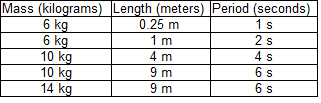According to the data presented, what is the apparent relationship between massand period?

The two variables have negative linear correlation

The two variables have a negative non-linear correlation

The two variables are not related.

The two variables have a positive non-linear correlation

The two variables have a positive linear correlation

The two variables are not related.

Explanation:

The correct answer is that they are not related. For this question, the most important row in the presented table is the last row, because it demonstrates that changing the mass of the bob had no effect on the period of the pendulum. Increasing the mass of the bob by 4 kilograms did not affect the pendulum's period. Note that this is done while keeping the length of the pendulum constant. Therefore, we conclude that there is no correlation between the mass of a pendulum's bob,, and the pendulum's period,. This is indeed true experimentally.

### Example Question #11 : How To Find Research Summary In PhysicsThe period of a simple pendulumis defined as the amount of time that it takes for a pendulum to swing from one end to the other and back. In studying the period of a simple pendulum, two students express their opinions.

Student 1: The period of a pendulum depends on two factors: the mass of the pendulum's bob (the object swinging at the end of the pendulum) and the length of the pendulum. The height at which the pendulum is originally dropped does not affect the period.

Student 2: The period of a pendulumonly depends on the length of the pendulum. Varying the mass and the height at which the pendulum is originally dropped does not affect how long the pendulum takes to swing across.

The two students ran a series of trials to measure the period of a simple pendulum using varying weights and lengths. The students did not measure height as a factor. The results of the trials can be seen in the table below:According to the data, what is the apparent relationship between lengthand period?

The two variables have a negative linear correlation.

There is no apparent relationship.

The two variables have a positive linear correlation.

The two variables have a negative non-linear correlation.

The two variables have a positive non-linear correlation.

The two variables have a positive non-linear correlation.

Explanation:

The correct answer is that they are positively and non-linearly related. We can ignore the change in mass because the last two rows of the chart demonstrate that changing the mass of a pendulum's bob does not affect the period of the pendulum. As we can see in the chart, once we ignore the changing mass, we see that increasing the length by a factor of four only tends to increase the period by a factor of two. This shows a non-linear but still positive correlation. Although it wouldn't be hard to discern what exactly this non-linear relationship is, all we need for this question is to know that it is non-linear.

### Example Question #20 : Physics

A particle separator functions by using a magnetic field and an electric field pointing perpendicularly. When a charged particle is launched into the particle separator, a constant electric force is exerted on the particle proportional to the particle's charge. Additionally, the magnetic field exerts a force on the particle that is in the opposite direction of the electric force and that is proportional to the particle's charge and velocity. A particle will make it through the particle separator only if the opposing magnetic forces and electric forces are equal in magnitude as they will not cause a net change in the particle's direction.

What can we infer would happen if a proton (a particle with a positive charge) were shot into the particle accelerator with an extremely low speed?

The particle would be pushed in the direction of the electric force.

The particle would be pushed in the direction of the magnetic force.

The particle would accelerate.

The particle would slow to a stop.

The particle would eventually make it out of the particle separator.